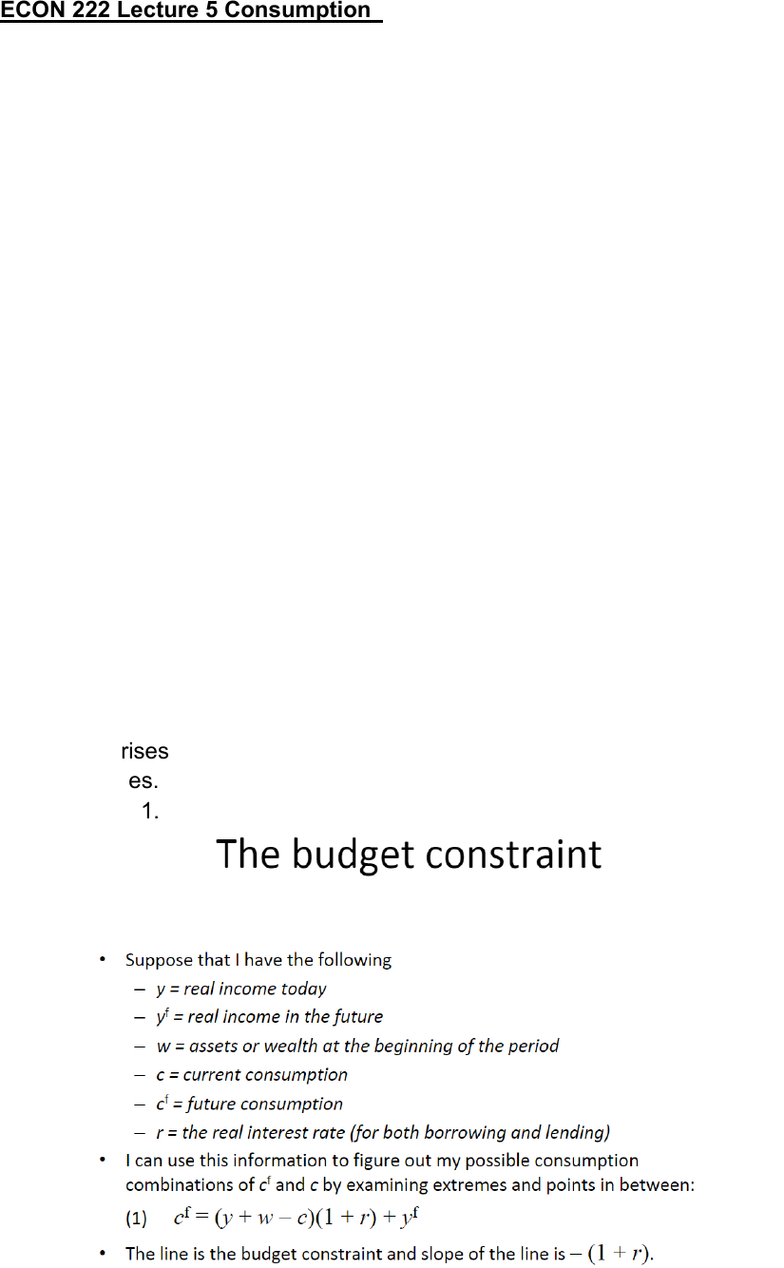Class Notes (1,100,000)
CA (650,000)
Queen's (10,000)
ECO (500)
Lecture 5

# ECON 222 Lecture Notes - Lecture 5: Real Interest Rate, Consumption SmoothingPremium

Department
Economics
Course Code
ECON 222
Professor
Mike Kennedy
Lecture
5

This preview shows half of the first page. to view the full 3 pages of the document.ECON 222 Lecture 5 Consumption
Consumption & Saving
Desired national consumption: C^d
Desired national saving: S^d
Recall for Chapter 2: If net factor payment (NFP)is zero, the national saving
S equals to Y-C-G (note: Y-output; C-consumption; G-government spending)
Therefore, we can deduce the following function: S^d=Y-C^d-G (note: S^d
is the total of private and government saving)
The consumption and saving decision of individual
Assume the real interest rate is r. Then for 1 dollar today will worth 1+r in
next time period.
If a person borrows 1 dollar this year, he has to pay 1+r dollar next time
period. Similarly, if that person saves 1 dollar this year, he can get 1+r dollar
next time period. Therefore, 1+r is the price of a dollar current consumption
is terms of future consumption.
*Two ways of choice making:
1. Borrow heavily and consume more than the person’s income today
2. Save nearly all of the income
What most people do?
Avoid periods of very high or very low consumption which is known as
consumption- smoothing motive.
Effect of changes in current income
Marginal propensity to consume (MPC):
The fraction of additional current income that is consumed in the current
period
When Y rises by 1, the C^d typically rise less than 1. S^d also increases as
Y increases. If the money does not spend on consumption, S^d rises by the
fraction of 1.
Prof. Kennedy, Lecture 5, Slide 11